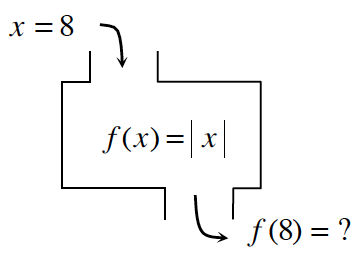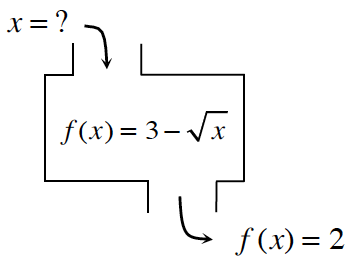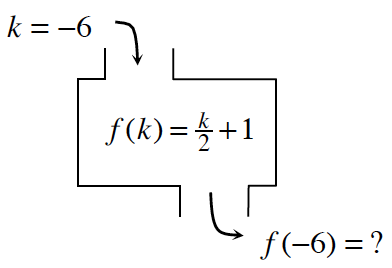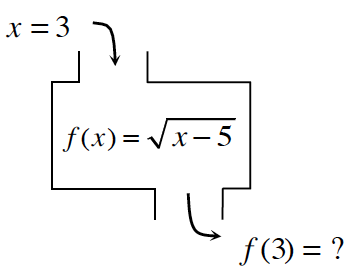### Home > AC > Chapter 11 > Lesson 11.1.5 > Problem11-57

11-57.

Find the corresponding inputs or outputs for the following relations. If there is no solution, explain why not. Be careful: In some cases, there may be no solution or more than one possible solution.

1.Substitute $x$ into the equation.

$f\left(8\right)=\left|8\right|$

1.Set up the equation.

$2=3-\sqrt{x}$

Solve for $x$.

$x=1$

1.Refer to part (a).

1.Refer to part (a).

no solution